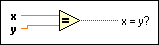# Equal? Function

FlexRIO Help

Edition Date: November 2015

Part Number: 372614J-01

»View Product Info

Owning Palette: Comparison Functions

Requires: Base Development System

Returns TRUE if x is equal to y. Otherwise, this function returns FALSE. You can change the comparison mode of this function.

If you compare two matrices, the default comparison mode is Compare Aggregates, and this function returns a scalar. You can compare an array or cluster of a data type to a scalar of the same data type and produce an array or cluster of Boolean values. The connector pane displays the default data types for this polymorphic function.x and y must be of the same type.When you compare arrays, x = y? is a scalar in Compare Aggregates mode and a Boolean array in Compare Elements mode (default).

## Equal? Details

When comparing VI Server references, this function returns TRUE when the two references refer to the same object, regardless of whether the references have the same capability or the same value. For example, one might be a strict reference to a digital numeric control and the other might be a generic reference but if they refer to the same object, they are equal. To compare the actual values of the references, use the Type Cast function to convert the references to 32-bit signed integers. Then use the Equal? function to compare those integers.

Similarly to the previous situation, if you have a local reference and a remote reference to the same LabVIEW object, this function returns FALSE. However, when two references refer to the same object but one of them is authenticated and the other is not, this function still returns TRUE.Note  If you compare two inputs with the value not a number, NaN, or one input with the value NaN to an input with another value, this function always returns FALSE. Use the Not a Number/Path/Refnum? function to compare one or more inputs with a value of NaN.

When you wire matrix data as an input to this function, a VI that includes subVIs that work with the matrix data type replaces the function. The resulting VI has the same icon but contains a matrix-specific algorithm. The node remains a VI if you disconnect the matrix from the input(s). Wire other data types as inputs to restore the original function. If you wire a data type to a function and that data type causes a basic math operation to fail, the function returns an empty matrix or NaN.

## Example

Refer to the Comparison Functions VI in the labview\examples\Comparison directory for an example of using the Equal? function.